# 用 JavaScript 写出一个彩票系统？

• 2023-03-22
上海
• 本文字数：9677 字

阅读完需：约 32 分钟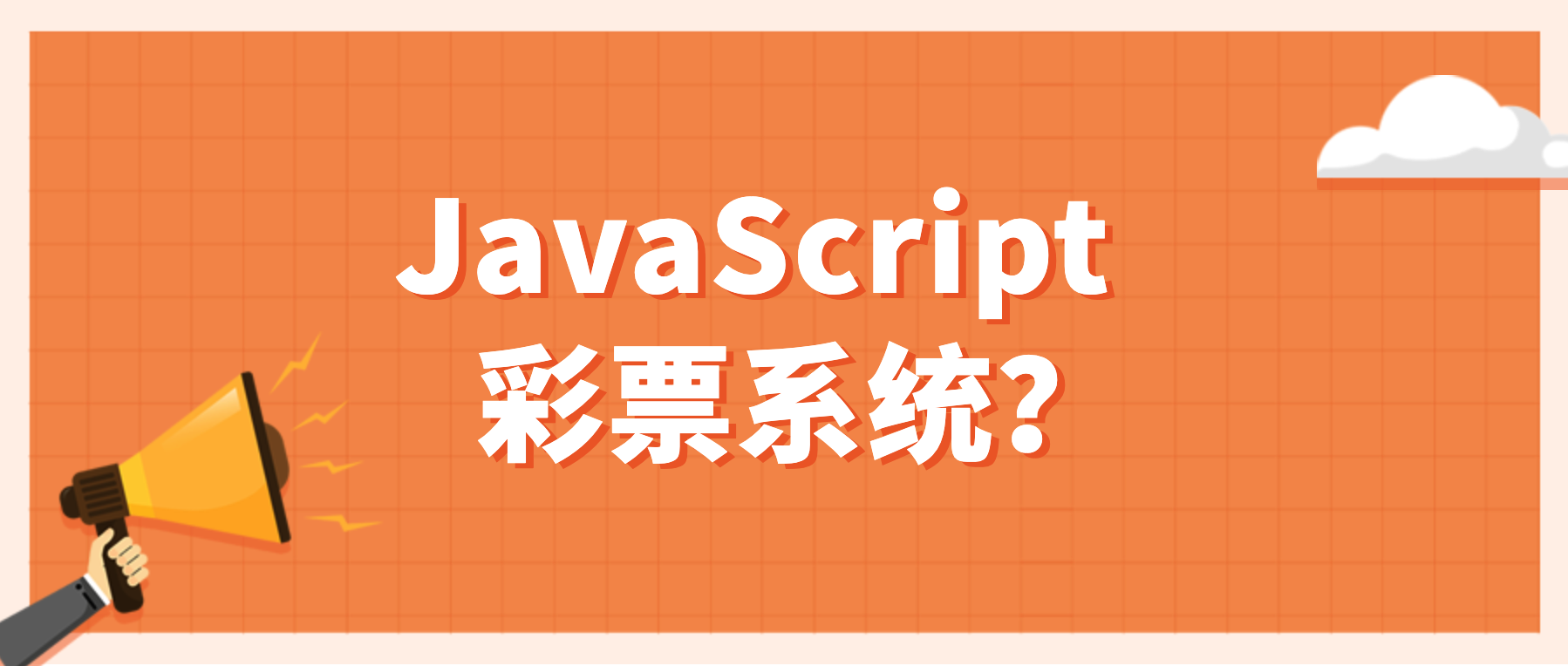## 杂谈

1. 获取历史以来所有的中奖彩票号码

2. 使用代码去统计出所有号码的中奖次数

3. 按照出现几率最低的数字来排序

4. 依次组成某几注新号码

## 彩票的规则

1. 红球为六位，选项从 1 - 33 中挑选，不可重复

2. 蓝球为一位，选项从 1 - 16 中挑选

3. 红蓝双色球一共七位组成一注

function factorial(n) {  if (n === 0 || n === 1) {    return 1  } else {    return n * factorial(n - 1)  }}function combination(n, k) {  return factorial(n) / (factorial(k) * factorial(n - k))}console.log(combination(33, 6) * combination(16, 1)) // 17721088

## 数据量

const totalSize = 17721088 * 14 / 1024 / 1024 // 236.60205078125MB

01这个数字在内存中的占用是两个字节，也就是 2B，那如果我们把 01 用小写 a 代替，那么其容量就可以变成 1B，总体容量可减少一半左右！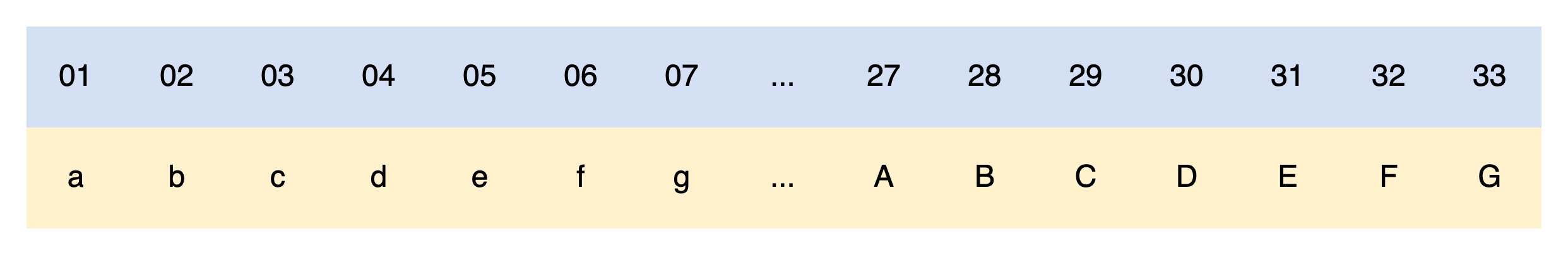• 有损压缩算法：这些算法能够在压缩数据时丢弃一些信息，但通常能在不影响实际使用的前提下实现更高的压缩比率，其中最常见的是图像、音频和视频压缩算法

• 无损压缩算法：这些算法不会丢弃任何信息，它们通过查找输入数据中的重复模式，并使用更短的符号来表示它们来实现压缩。无损压缩算法常用于文本、代码、配置文件等类型的数据

function generateCombinations(arr, len, maxCount) {  let result = []    function generate(current, start) {    // 如果已经生成的组合数量达到了最大数量，则停止生成    if (result.length === maxCount) {      return    }    // 如果当前已经生成的组合长度等于指定长度，则表示已经生成了一种组合    if (current.length === len) {      result.push(current)      return    }    for (let i = start; i < arr.length; i++) {      current.push(arr[i])      generate([...current], i + 1)      current.pop()    }  }  generate([], 0)  return result}

function getDoubleColorBall(count) {  // 红球数组：['01', '02' .... '33']  const arrRed = Array.from({ length: 33 }, (_, index) => (index + 1).toString().padStart(2, '0'))  const arrRedResult = generateCombinations(arrRed, 6, count)  const result = []  let blue = 1  arrRedResult.forEach(line => {    result.push(line.join('') + (blue++).toString().padStart(2, '0'))    if (blue > 16) {      blue = 1    }  })  return result}

const firstPrize = getDoubleColorBall(1000).join('')fs.writeFileSync('./hello.txt', firstPrize)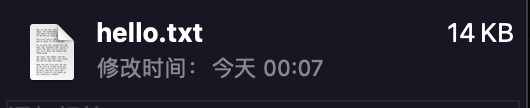function compressHello() {  const letters = 'abcdefghijklmnopqrstuvwxyzABCDEFG'  const doubleColorBallStr = getDoubleColorBall(1000).join('')  let resultStr = ''  for (let i = 0; i < doubleColorBallStr.length; i+=2) {    const number = doubleColorBallStr[i] + doubleColorBallStr[i+1]    resultStr += letters[parseInt(number) - 1]  }  return resultStr}const firstPrize = compressHello()fs.writeFileSync('./hello-1.txt', firstPrize)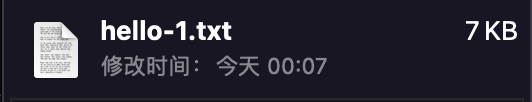## 更精妙的方法

• 具备数据不丢失的特性，也就是无损压缩

• 压缩率尽可能小，因为传输的文件可能非常大，如我们上面举的例子

• 便于信息的传输，也就是支持 HTTP 请求

const zlib = require('zlib')const firstPrize = compressHello()fs.writeFileSync('./hello-2.txt.gz', zlib.gzipSync(firstPrize))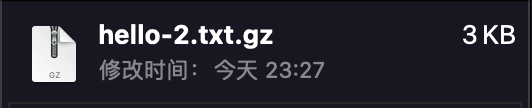content-encoding 响应头一般是服务器针对返回的资源响应编码格式的设置信息，常见的值有以下三种：

• gzip 所有浏览器都支持的通用压缩格式

• brotligzip 压缩性能更好，压缩率更小的一个新的压缩格式，老版本浏览器不支持

• deflate 出于某些原因，使用不是很广泛，后有基于该算法的 zlib 压缩格式，不过也使用度不高

const firstPrize = compressHello()fs.writeFileSync('./hello-2.txt.gz', zlib.gzipSync(firstPrize))fs.writeFileSync('./hello-2.txt.def', zlib.deflateSync(firstPrize))fs.writeFileSync('./hello-2.txt.br', zlib.brotliCompressSync(firstPrize))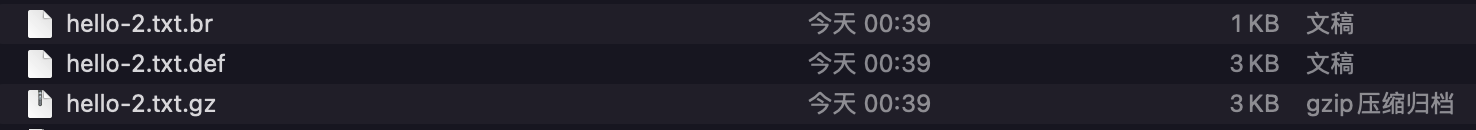// 执行解压操作const brFile = fs.readFileSync('./hello-2.txt.br')const gzipFile = fs.readFileSync('./hello-2.txt.gz')const deflateFile = fs.readFileSync('./hello-2.txt.def')const brFileStr = zlib.brotliDecompressSync(brFile).toString()const gzipFileStr = zlib.gunzipSync(gzipFile).toString()const deflateFileStr = zlib.inflateSync(deflateFile).toString()console.log(brFileStr)console.log(gzipFileStr)console.log(deflateFileStr)console.log(brFileStr === gzipFileStr, brFileStr === deflateFileStr) // true, true

## 完整的数据

function generateAll() {  const arrRed = Array.from({ length: 33 }, (_, index) => (index + 1).toString().padStart(2, '0'))  const arrRedResult = generateCombinations(arrRed, 6, Number.MAX_VALUE)  const result = []  arrRedResult.forEach(line => {    for (let i = 1; i <= 16; i++) {      result.push(line.join('') + i.toString().padStart(2, '0'))    }  })  return result}console.log(generateAll().length) // 17721088

function compressAll() {  const letters = 'abcdefghijklmnopqrstuvwxyzABCDEFG'  const allStr = generateAll().join('')  let resultStr = ''  for (let i = 0; i < allStr.length; i += 2) {    const number = allStr[i] + allStr[i+1]    resultStr += letters[parseInt(number) - 1]  }  return resultStr}const firstPrize = compressAll()fs.writeFileSync('./all-ball.txt', firstPrize)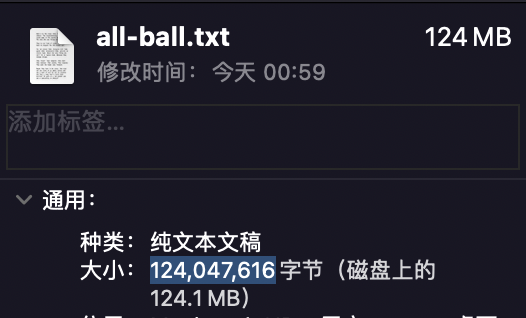const totalSize = 124047616 / 1024 / 1024 // 118.30102539 MB

const firstPrize = compressAll()fs.writeFileSync('./all-ball.txt.gz', zlib.gzipSync(firstPrize))fs.writeFileSync('./all-ball.txt.br', zlib.brotliCompressSync(firstPrize))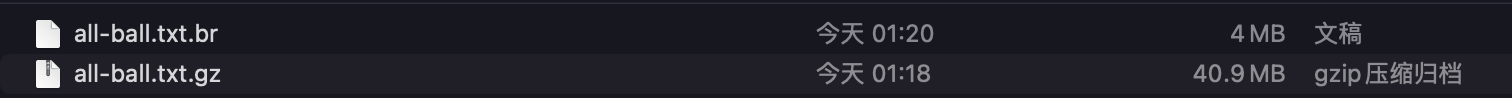## 随机来两注

• 什么时候进行分发

• 数据回源如何做

• 如何避免所有数据被劫持

• 数据交给彩票站的策略

### 什么时候进行分发

1. 从目前已有的彩票库里面，按照号码出现几率从高到低排列

2. 挑选出前 50 万注分发给 30 万彩票站，这个时间彩票站的数据都是统一的

3. 每个小时同步一次数据，同步的是其他彩票站"特意挑选的数据"

50 万注的数据量有多大？试试看：

function getFirstSend() {  const letters = 'abcdefghijklmnopqrstuvwxyzABCDEFG'  const doubleColorBallStr = getDoubleColorBall(500000).join('')  let resultStr = ''  for (let i = 0; i < doubleColorBallStr.length; i+=2) {    const number = doubleColorBallStr[i] + doubleColorBallStr[i+1]    resultStr += letters[parseInt(number) - 1]  }  return resultStr}const firstPrize = getFirstSend()fs.writeFileSync('./first-send.txt.br', zlib.brotliCompressSync(firstPrize))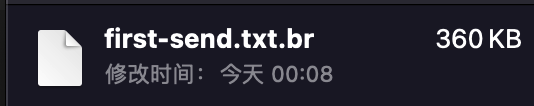function decodeData(brFile) {  const result = []  const content = zlib.brotliDecompressSync(brFile)  // 按照七位每注的结构拆分  for (let i = 0; i < content.length; i += 7) {    result.push(content.slice(i, i + 8))  }  return result}const firstSend = fs.readFileSync('./first-send.txt.br')const firstDataList = decodeData(firstSend)console.log(firstDataList.length) // 500000

function letterToCode(letterStr) {  const result = []  const letters = 'abcdefghijklmnopqrstuvwxyzABCDEFG'  for (let i = 0; i < letterStr.length; i++) {    result.push((letters.indexOf(letterStr[i]) + 1).toString().padStart(2, '0'))  }  return result}

• 低性能：TPS 在 50 以下，适用于低流量的应用场景，例如个人博客、小型企业网站等。

• 中性能：TPS 在 50~500 之间，适用于一般的网站和应用场景，例如中小型电商网站、社交网络等。

• 高性能：TPS 在 500~5000 之间，适用于高流量的网站和应用场景，例如大型电商网站、游戏网站等。

• 超高性能：TPS 在 5000 以上，适用于超高流量的网站和应用场景，例如互联网巨头的网站、在线游戏等。

### 数据回源如何做

// 服务器集群数量const machineCount = 100// 总访问量，50%中的号码才会上报const totalVisit = 50000000 * 0.5 // 25000000// 总的时间，因为我们计算的是 10个小时的时间，所以应该计算的总秒数为 36000 秒！const totalSeconds = 10 * 60 * 60console.log(totalVisit / totalSeconds / machineCount) // 6.944444444444445

TPS 仅为 7！！这还是没有排除掉已经知悉的号码的情况，具体的上报逻辑，参考下图：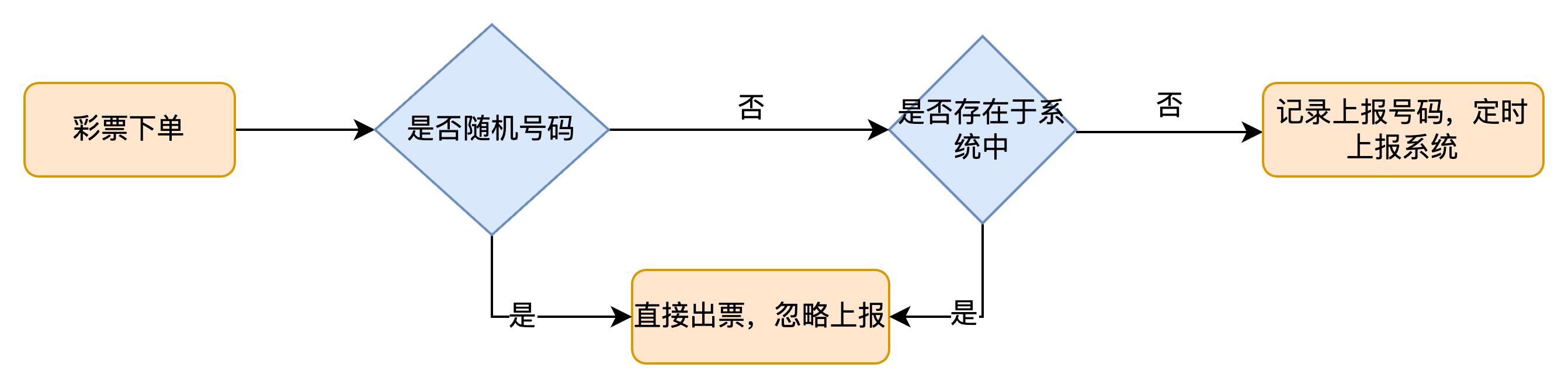### 数据交给彩票站的策略(避免数据被劫持)

• 数据同步难度，跨地区同步对服务器压力巨大，如华南向华北同步

• 数据相似程度，两地的数据如果历史以来相似度区别很大，反而不能达到覆盖的目的，因为我们最终是想要这注号码被购买更多次

• 数据同步时差，如新疆等地，鉴于网络问题，比其他地要慢很多的情况，这样就会漏号，那么就应该把这些地方的数据同步到更繁华的区域，如上海市，但是这一点看似是和第一二点相悖的

function random(count) {  let result = []  for (let i = 0; i < count; i++) {    const index = Math.floor(Math.random() * firstDataList.length)    console.log(firstDataList[index])    result.push(letterToCode(firstDataList[index]))  }  return result}console.log(random(2))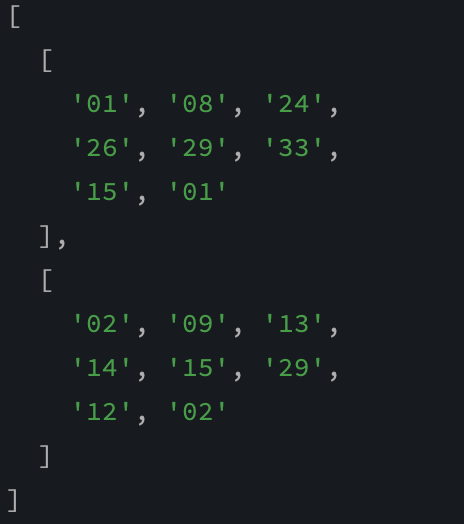OK，你觉得可以中奖吗？哈哈哈，还是有可能的，继续往下看吧！

## 我要一等奖

1. 6 + 1 一等奖 奖金 500 万

2. 6 + 0 二等奖 奖金 30 万

3. 5 + 1 三等奖 奖金 3000 元

4. 5 + 0 或 4 + 1 四等奖 奖金 200 元

5. 4 + 0 或 3 + 1 五等奖 奖金 10 元

6. 2 + 1 或 1 + 1 或 0 + 1 都是 六等奖 奖金 5 元

/** * @param {String[]} target ['01', '02', '03', '04', '05', '06', '07'] * @param {String[]} origin ['01', '02', '03', '04', '05', '06', '07'] * @returns {Number} 返回当前彩票的中奖金额 */function compareToMoney(target, origin) {  let money = 0  let rightMatched = target === origin  // 求左边六位的交集数量  let leftMatchCount = target.slice(0, 6).filter(    c => origin.slice(0,6).includes(c)  ).length  if (leftMatchCount === 6 && rightMatched) {    money += 5000000  } else if (leftMatchCount === 6 && !rightMatched) {    money += 300000  } else if (leftMatchCount === 5 && rightMatched) {    money += 3000  } else if (leftMatchCount === 5 && !rightMatched) {    money += 200  } else if (leftMatchCount === 4 && rightMatched) {    money += 200  } else if (leftMatchCount === 4 && !rightMatched) {    money += 10  } else if (leftMatchCount === 3 && rightMatched) {    money += 10  } else if (leftMatchCount === 2 && rightMatched) {    money += 5  } else if (leftMatchCount === 1 && rightMatched) {    money += 5  } else if (rightMatched) {    money += 5  }  return money}

• 随机生成一组中奖号码

• 对于每个购买的数字，检查是否与中奖号码匹配，并计算它的奖金金额

• 对于所有购买的数字的奖金金额进行求和

• 重复这个过程，直到找到最优的中奖号码

• 将所有的号码按照购买数量进行排序（其实这里真实的场景应该联合考虑中奖号码的分布趋势才更精确）

• 从空号开始查询，依次进行计算

function getRandomCode(count = 500000) {  const arrRed = Array.from({ length: 33 }, (_, index) => (index + 1).toString().padStart(2, '0'))  // generateCombinations 是我们上面定义过的函数  const arrRedResult = generateCombinations(arrRed, 6, count)  const result = []  let blue = 1  arrRedResult.forEach(line => {    result.push([...line, (blue++).toString().padStart(2, '0')])    if (blue > 16) {      blue = 1    }  })  return result}function randomPurchase() {  const codes = getRandomCode()  const result = []  for (let code of codes) {    let count = Math.floor(Math.random() * 50)    result.push({      code,      count,    })  }  return result}console.log(randomPurchase())

[  {    code: [      '01', '02',      '03', '04',      '05', '10',      '05'    ],    count: 17  },  {    code: [      '01', '02',      '03', '04',      '05', '11',      '06'    ],    count: 4  }]

// 空号在前，购买数量越多越靠后const purchaseList = randomPurchase().sort((a, b) => a.count - b.count)const bonusPool = []for (let i = 0; i < purchaseList.length; i++) {  // 假设这就是一等奖，那么就需要计算其价值  const firstPrize = purchaseList  let totalMoney = 0  for (let j = 0; j < purchaseList.length; j++) {    // 与一等奖进行对比，对比规则是参照彩票中奖规则    const money = compareToMoney(purchaseList[j].code, firstPrize.code) * purchaseList[j].count    totalMoney += money  }  bonusPool.push({    code: firstPrize.code,    totalMoney,  })}const result = bonusPool.sort((a, b) => a.totalMoney - b.totalMoney)// 至于怎么挑，那就随心所欲了console.log(result.code, result.totalMoney)

## 结语### 泰罗凹凸曼

https://www.github.com/taroxin

## 评论